Power Torque continues to be a leading integrator in the motion control and electrical / mechanical power transmission fields. Let our combined experience of over 400 years design a solution for you.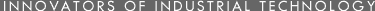• •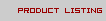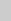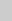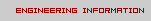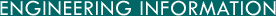H I J K L M

H

• Hand
= 4 inches, 10.160 centimeters.

• Hectare
= 2.471044 acres, 2.471058 Br acres, 395.367 square rods, 1.195985x10^4 square yards, 1.0764x10^5 square feet, 100 ares, 1x10^4 square meters.

• Hectogram
= 3.52739 avoirdupois ounces, 100 grams.

• Hectoliter
= 2.8378 bushels, 2.7497 Br bushels, 11.3513 pecks, 100 liters.

• Hectometer
= 19.8838 rods, 109.3611 yards, 109.3614 Br yards, 328.08 feet, 100 meters.

• Hectowatt
= 100 watts.

• Hemisphere
= 0.5 sphere, 4 spherical right angles, 6.2832 steradians.

= 63 Br gallons, 10.114 cubic feet, 0.28640 cubic meters.

= 63 gallons, 8.4218 cubic feet, 0.23848 cubic meters.

• Horsepower
= 0.70696 BTU (mean), 0.7452 kilowatt (g=980), 0.74570 kilowatt (g=980.665), 1.0139 horse power (metric) or cheval-vapeur, 10.688 kilogram-calories (mean) per minute, 42.418 BTU (mean) per minute, 550 foot-pounds per second, 745.2 watts (g=908), 745.70 watts (g=980.665), 3.3000x10^4 foot-pounds per minute.

• Horsepower, electrical (US and BR)
= 746.00 watt (absolute) commonly used in rating electrical machinery.

• Horsepower, metric (cheval vapeur)
= 0.98632 horsepower (US), 75 kilogram-meters per second, 735.399 watts, 3.2549x10^4 foot-pounds per minute.

• Horsepower-hour
= 0.7457 kilowatt-hour, 641.3 kilogram-calories (mean), 745.7 watt-hours, 2545.0 BTU (mean), 2.7374x10^5 kilogram-meters, 1.9800x10^6 foot-pounds, 2.6845x10^6 joules (absolute).

• Horsepower-hour (electrical, US and BR)
= 2.6856x10^6 joules (absolute).

• Hour (tropical mean solar)
= 0.0059524 week, 0.041667 mean solar day, 60 mean solar minutes, 3600 mean solar seconds.

• Hundredweight (short)
= 100 pounds, 0.44643 long ton, 0.05 short ton, 4 Br quarters, 1600 avoirdupois ounces, 0.0453592 metric ton, 45.3592 kilograms.

• Hundredweight (long)
= 112 pounds, 0.05 long ton, 4 Br quarters, 50.8023 kilograms.

I

• Inch (US)
= 1.57828x10^-5 mile, 0.00126263 Gunter's chain, 0.00505051 rod, 1/36 yard, 1/12 foot, 0.126263 Gunter's link, 72 points (printer's type), 1000 mils, 2.540005 centimeters, 25.40005 millimeters, 2.54x10^8 Angstrom unit, 39450.33 wave lengths of red line of cadmium.

• Inch (Br)
= 1/36 Br yard, 1/9 Br linear quarter, 2.539998 centimeters, 25.39998 millimeter.

• Inch (Paris)
= 1 Pouce.

• Inch of Hg (pressure) at 32 degrees F
= 0.033421 atmosphere, 0.49116 pound per square inch, 1.13299 feet of water at 39.1 degrees F, 13.595 inches of water at 4 degrees C, 70.727 pounds per square foot, 345.31 kilograms per square meter, 3.38639x10^4 dynes per square centimeter.

• Inch of water (pressure) at 4 degrees C
= 0.0024583 atmosphere, 0.036136 pounds per square inch, 0.07 3554 inch of Hg, 0.57818 ounce per square inch, 5.2022 pounds per square foot, 25.399 kilograms per square meter, 2490.82 dynes per square centimeter.

J

• Joule (absolute)
= 2.778x10^-7 kilowatt-hour, 3.725x10^-7 horse power-hour, 2.3889x10^-4 kilogram-calorie (mean), 2.778x10^-4 watt-hour, 3.485x10^-4 cubic foot-atmosphere, 9.480x10^-4 BTU (mean), 0.009869 liter-atmosphere, 0.10197 kilogram-meter, 0.23889 gram-calorie (mean), 0.23895 gram-calorie at 15°C, 0.23918 gram-calorie at 20°C, 0.73756 foot-pound; 0.999680 joule (Int'l), 1 watt-second, 1 newton-meter, 23.730 foot-poundals, 1.0197x10^4 gram-centimeters; 1x10^7 ergs, 1x10^7 dyne-centimeter.

• Joule (Int'l)
= 1.00032 joule (absolute).

K

• Kilderkin
= 18 Br gallons, 0.081830 cubic meter.

• Kilogram
= 9.842064x10^-4 long ton, 0.0011023112 short ton, 0.001 metric ton, 0.019684 long hundredweight, 0.022046223 short hundredweight, 0.07874 Br quarter, 2.2046223 avoirdupois pounds, 2.6792285 apothecary or troy pounds, 32.150742 apothecary or troy ounces, 35.273957 avoirdupois ounces, 257.21 apothecary or troy dram, 564.38 avoirdupois dram, 643.01 pennyweight, 771.62 scruples, 1.54324x10^4 grains, 1000 grams.

• Kilogram-calorie or large calorie (mean)
= 0.0011628 kilowatt-hour, 0.0015593 horse power-hour, 1.1628 watt-hour, 3.9685 BTU (mean), 426.85 kilogram-meters, 1000 small or gram-calories, 3087.4 foot-pounds, 4186 joules, 4.2686x10^7 gram-centimeters, 4.186x10^10 ergs.

• Kilogram-meter
= 2.7235x10^-6 kilowatt hour, 3.6530x10^-6 horse power-hour, 0.0027235 watt-hour, 0.0034177 cubic foot-atmosphere, 0.0092972 BTU (mean), 0.096782 liter-atmosphere, 2.3427 gram-calories (mean), 7.2330 foot-pounds, 9.80665 joules (absolute); 232.71 foot-poundals, 1x10^5 gram-centimeters, 9.80665x10^7 ergs.

• Kiloliter
= 1.3080 cubic yards, 35.316 cubic feet, 264.18 gallons, 1.000027 cubic meters, 1000 liters.

• Kilometer
= 1.0567x10^-13 light year, 0.53996 nautical mile, 0.62137 statute mile, 1093.6 yards, 3280.8 feet, 0.1 myriameter, 1000 meters, 1x10^5 centimeters.

• KiloPascal
= 9.869x10^-3 Standard Atmospheres, 0.14515 psi.

• Kilowatt
= 0.23889 kilogram-calorie (mean) per second, 0.94827 BTU (mean), 1.3410 horsepower, 1.3597 horse power (metric), 14.333 kilogram-calories (mean) per minute, 56.896 BTU (mean) per minute, 737.56 foot-pounds per second, 1000 watts, 2.6552x10^6 foot-pounds per hour.

• Kilowatt-hour
= 1.3410 horse power-hours; 1000 watt-hours, 3413.0 BTU (mean), 3.6710x10^5 kilogram-meters, 8.6001x10^5 gram-calories (mean) 2.6552x10^6 foot-pounds, 3.6000x10^6 joules (absolute).

• Knot (as a unit of length)
= 1 nautical mile.

• Knot (velocity)
= 1 nautical mile per hour, 1.1516 miles per hour, 1.689 feet per second, 1.853 kilometers per hour, 51.48 centimeters per second., 6080.20 feet per hour.

L

• Large calorie
= See kilogram-calorie.

• Last (Br)
= 80 bushels (variable), 2.9095 cubic meters.

• League (statute)
= 3 statute miles, 4.8280 kilometers.

• League (nautical)
= 3 nautical miles, 5.5560 kilometers.

• Light (speed of)
= 2.9986x10^10 centimeters per second.

• Light Year
= 5.9x10^12 miles, 9.4637x10^12 kilometers.

• Ligne (Paris line)
= 1/12 pouce or Paris inch, 0.22583 centimeter.

• Line (Br)
= 1/12 inch, 0.21167 centimeters.

= 0.01 Engineer's chain, 1 foot, 12 inches, 30.480 centimeters.

= 1.25x10^-4 miles, 0.01 Gunter's chain, 0.04 rod, .22 yards, 0.66 foot, 7.92 inches, 0.2011684 meter, 20.11684 centimeter.

• Liter
= 0.0013080 cubic yard, 0.028378 bushel, 0.027497 Br bushel, 0.26417762 gallon, 0.21998 Br gallon, 0.035316 cubic feet, 0.11351 peck, 0.10999 Br peck, 0.908102 dry quart, 1.056710 liquid quarts, 0.87990 Br quart, 1.8162 dry pints, 2.1134 liquid pints, 1.7598 Br pints, 8.4538 gills, 7.0392 Br gills, 33.8147 fluid ounces, 35.196 Br fluid ounces, 61.025 cubic inches, 270.5179 fluid drams, 0.001000027 cubic meter, 1.000027 cubic decimeter, 1000.027 cubic centimeter.

• Liter-atmosphere (normal)
= 3.7745x10^-5 horse power-hour, 0.035319 cubic foot-atmosphere, 0.09607 BTU (mean), 10.333 kilogram-meters, 24.206 gram-calories (mean), 74.735 foot-pounds, 101.328 joules (absolute), 2404.5 foot-poundals.

• Liter-atmosphere (lat. 45°, g=980.616)
= 101.323 joules (absolute).

• La livre (French Pound)
= 0.5 kg.

• Lumen
= 0.001496 watt.

M

• Mean Solar Day
= 1440 minutes, 86,400 seconds.

• Megalerg
= 1x10^6 ergs.

• Megameter
= 1x10^6 meter.

• Meter
= 5.3996x10^-4 nautical mile, 6.2137x10^-4 statute mile, 0.00497096 furlong, 0.0497096 Gunter's chain, 0.198838 rod, 0.54681 fathom, 1.093611 yard, 1.093614 Br yard, 3.280833 feet, 3.280843 Br feet, 3.0784 Fr pied, 4.970960 Gunter's links, 39.3700 inches, 39.3701 Br inches, 1x10^06 megameter, 0.001 kilometer, 100 centimeters, 1x10^9 millimicrons (micromillimeter), 1x10^10 angstrom units, 1x10^12 millionth microns, 1.55316413x10^6 wavelength of red line of cadmium.

• Metric Ton
= 1 tonne, 1 millier.

• Microgram
= 1x10^-6 gram, 0.001 milligram.

• Microliter
= 1x10^-6 liter.

• Micromicron
= 1x10^-12 meter.

= 0.206265 seconds.

• Micron
= 3.937x10^-5 inch, 0.039370 mil, 39.37 millionths of an inch, 1x10^-6 meter, 1x10^-4 centimeter, 0.001 millimeter, 1000 milli-microns (micro-millimeters), 1x10^4 Angstrom units.

• Mil
= 0.001 inch, 0.00254001 centimeter, 0.0254001 millimeter, 25.4001 microns.

• Mil (Angular)

• Mil (Military)
= 360/6400 degrees.

• Mile (statute)
= 1.69x10^-13 light year, 0.86898 nautical mile, 8 furlongs, 80 Gunter's chains, 320 rods, 1760 yards, 5280 feet, 8000 Gunter's links, 6.3360x10^4 inches, 0.160935 myriameters, 1.60935 kilometers, 1609.35 meters.

• Mile (nautical)
= The length of 1 minute of arc on the earth's surface at the equator, 1/3 league, 1.15078 statute miles, 2025.37 yards, 6076.12 feet, 1.8520 kilometers.

• Mile (British)
= 1.60934 kilometers.

• Millier
= 1 tonne, 1 metric ton.

• Milligram
= 2.2046x10^-6 avoirdupois pound, 2.67923x10^-6 apothecary or troy pound, 3.52739x10^-5 avoirdupois ounce, 3.215074x10^-5 apothecary or troy ounce, 5.64383x10^-4 avoirdupois dram, 2.57206x10^-4 apothecary or troy dram, 6.43015x10^-4 pennyweight, 7.71618x10^-4 scruple, 0.01543236 grains, 1x10^-6 kilogram, 0.001 gram, 0.005 metric carat.

• Milliliter
= 0.0084538 gill, 0.0338147 fluid ounce, 0.035196 fluid Br ounce, 0.061025 cubic inch, 0.2705179 fluid dram, 16.2311 minims, 0.001 liter, 1.000027 cubic centimeter.

• Millimeter
= 0.0393700 inch, 0.0393701 Br inch, 39.37 mils, 0.001 meter, 0.1 centimeter, 1000 microns.

• Milli-micron
= 1 micro-millimeter, 1x10^-9 meter, 1x10^-7 centimeter, 0.001 micron, 10 Angstrom units.

• Millionth Micron
= 1 micromicron, 1x10^-12 meter, 1x10^-10 centimeter, 0.01 Angstrom units.

• Miner's Inch
= 1.5 cubic feet per minute or 11.25 GPM (in AZ CA MT NV OR), 1.2 cubic feet per minute or 9.0 GPM (in ID KS NE NM ND SD UT).

• Minim (fluid US)
= 1/61440 gallon, 1.3021x10^-4 liquid pint, 5.2083x10^-4 gill, 1/480 fluid ounce, 1/60 fluid dram, 0.061610 milliliter, 0.061612 cubic centimeter, 61.612 cubic millimeter.

• Minim (Br)
= 0.059194 cubic centimeter.

• Minute (angle)

• Minute (time)
= 9.9206x10^-5 week, 6.94446x10^-4 days, 0.016667 hour, 60 seconds.

• Month (mean calendar)
= 30.42 days, 730 hours, 4.38x10^4 minutes, 2.628x10^6 seconds.

• Month (lunar)
= 29 days 12 hours 44 minutes.

• Myriagram
= 22.04622 avoirdupois pounds, 10 kilograms, 1x10^4 grams.